# Multiple-group Chord diagram

From verion 0.4.10 of the circlize package, there is a new group argument in chordDiagram() function which is very convenient for making multiple-group Chord diagrams.

I first generate a random matrix where there are three groups (A, B, and C). Note this new functionality works the same for the input as a data frame.

library(circlize)
mat1 = matrix(rnorm(25), nrow = 5)
rownames(mat1) = paste0("A", 1:5)
colnames(mat1) = paste0("B", 1:5)

mat2 = matrix(rnorm(25), nrow = 5)
rownames(mat2) = paste0("A", 1:5)
colnames(mat2) = paste0("C", 1:5)

mat3 = matrix(rnorm(25), nrow = 5)
rownames(mat3) = paste0("B", 1:5)
colnames(mat3) = paste0("C", 1:5)

mat = matrix(0, nrow = 10, ncol = 10)
rownames(mat) = c(rownames(mat2), rownames(mat3))
colnames(mat) = c(colnames(mat1), colnames(mat2))
mat[rownames(mat1), colnames(mat1)] = mat1
mat[rownames(mat2), colnames(mat2)] = mat2
mat[rownames(mat3), colnames(mat3)] = mat3
mat
##        B1     B2    B3    B4     B5     C1    C2     C3     C4    C5
## A1  1.799  0.647 -1.35  0.23  1.340  0.045 -1.03 -0.001  1.667 -0.33
## A2  0.317  0.931  1.58 -0.33 -1.980  0.104 -2.29 -1.650 -1.065 -0.50
## A3  0.774  1.090  0.55 -0.52 -1.415  0.616  1.09  0.873 -0.310 -0.62
## A4  0.061  0.036  0.87 -0.90  0.024 -0.224  1.58 -0.782 -0.035  0.44
## A5 -0.262 -0.413  0.40  0.13  1.113  0.542  1.05  1.045  1.147  2.60
## B1  0.000  0.000  0.00  0.00  0.000 -1.433 -1.22  0.065  1.444  0.41
## B2  0.000  0.000  0.00  0.00  0.000 -1.035 -0.38 -0.413 -1.425  1.31
## B3  0.000  0.000  0.00  0.00  0.000 -0.942  0.51  0.137  0.017 -0.65
## B4  0.000  0.000  0.00  0.00  0.000  0.142 -1.12 -0.272  0.960  0.22
## B5  0.000  0.000  0.00  0.00  0.000 -1.247  1.66 -0.204 -0.986  0.16

The main thing is to create “a grouping variable”. The variable contains the group labels and the sector names are used as the names in the vector.

nm = unique(unlist(dimnames(mat)))
group = structure(gsub("\\d", "", nm), names = nm)
group
##  A1  A2  A3  A4  A5  B1  B2  B3  B4  B5  C1  C2  C3  C4  C5
## "A" "A" "A" "A" "A" "B" "B" "B" "B" "B" "C" "C" "C" "C" "C"

Assign group variable to the group argument:

grid.col = structure(c(rep(2, 5), rep(3, 5), rep(4, 5)),
names = c(paste0("A", 1:5), paste0("B", 1:5), paste0("C", 1:5)))
chordDiagram(mat, group = group, grid.col = grid.col)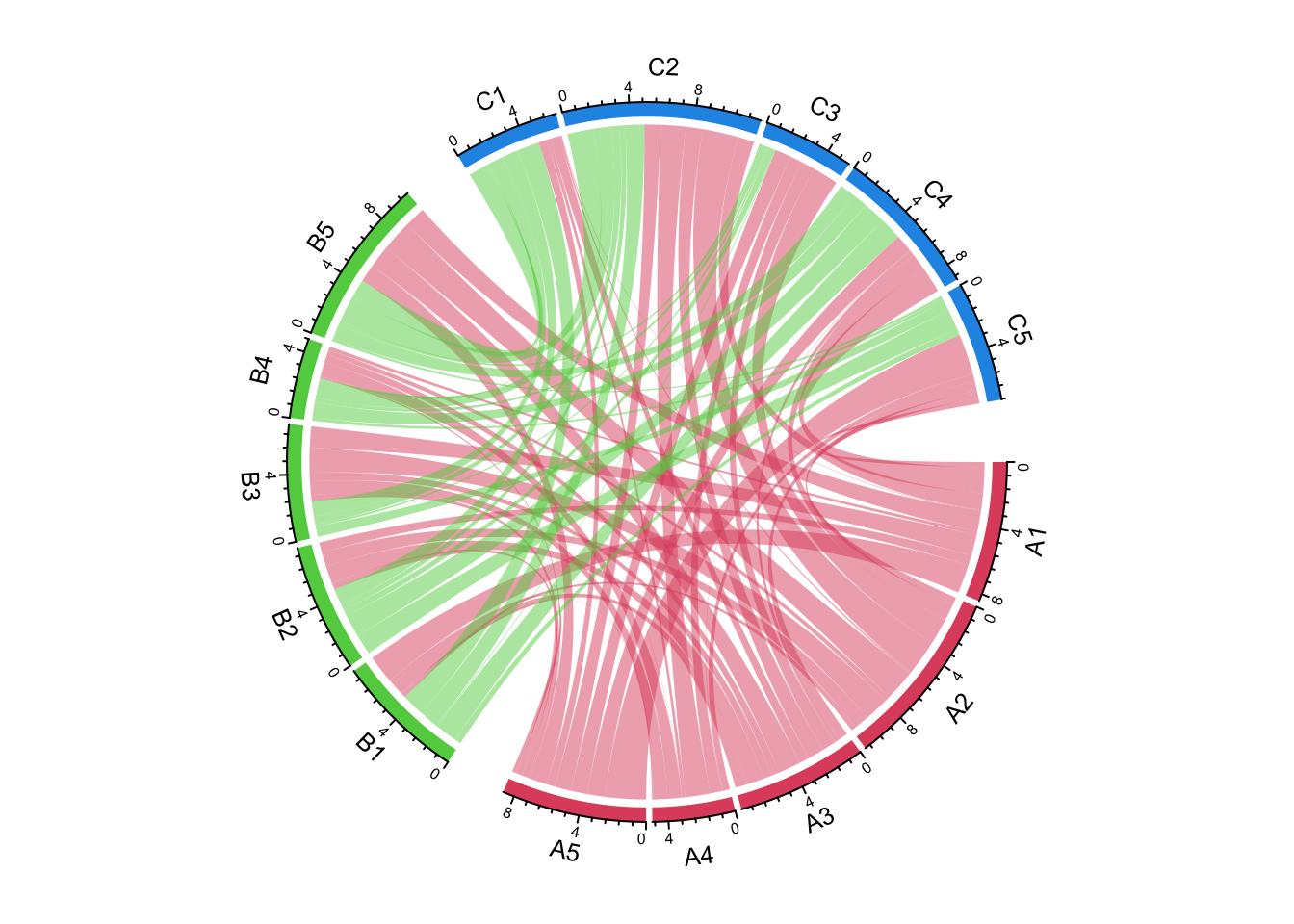circos.clear()

We can try another grouping:

group = structure(gsub("^\\w", "", nm), names = nm)
group
##  A1  A2  A3  A4  A5  B1  B2  B3  B4  B5  C1  C2  C3  C4  C5
## "1" "2" "3" "4" "5" "1" "2" "3" "4" "5" "1" "2" "3" "4" "5"
chordDiagram(mat, group = group, grid.col = grid.col)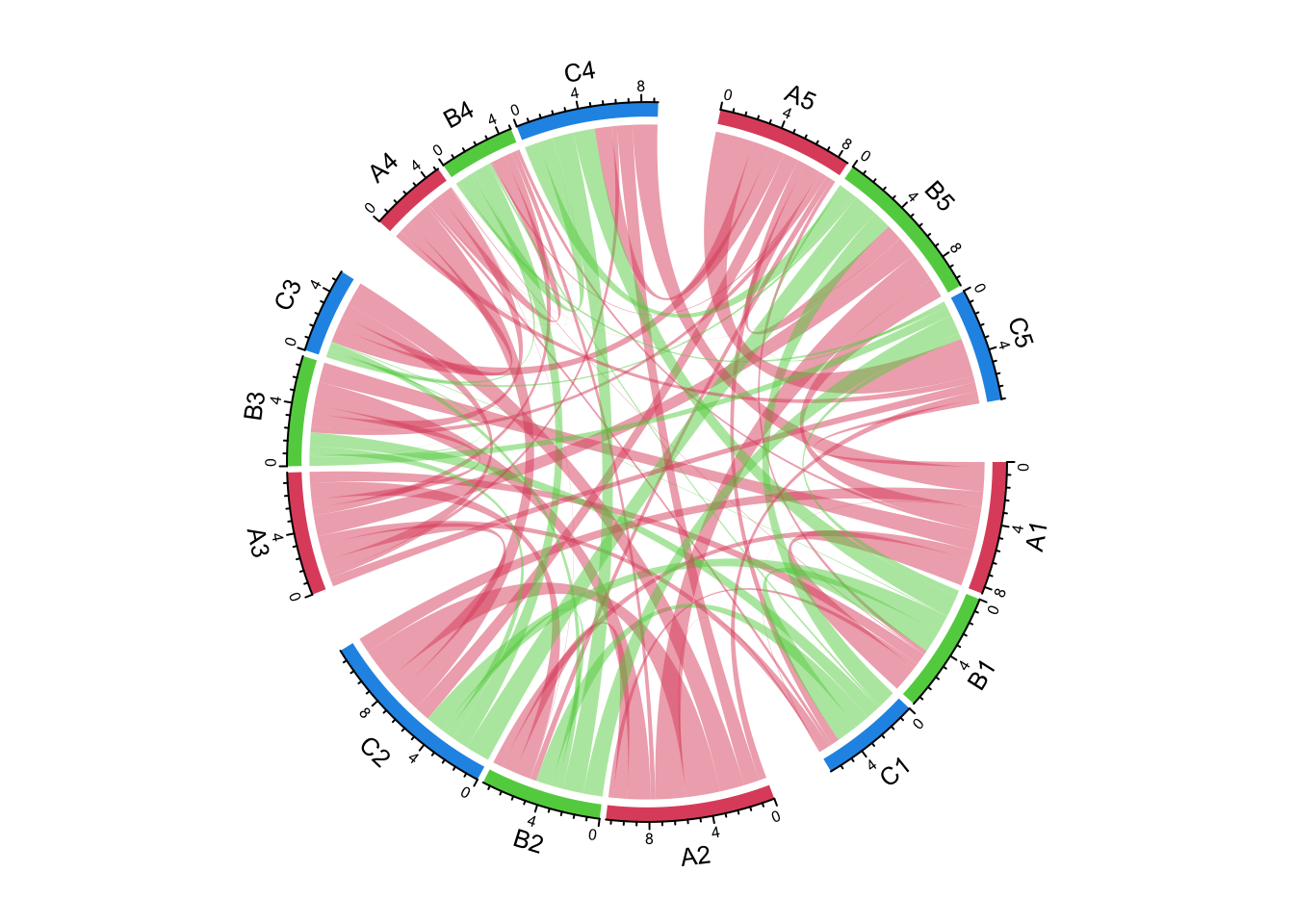circos.clear()

The order of group controls the sector orders and if group is set as a factor, the order of levels controls the order of groups.

group = structure(gsub("\\d", "", nm), names = nm)
group = factor(group[sample(length(group), length(group))], levels = c("C", "A", "B"))
group
## B2 C1 A5 A2 A1 C4 B5 C5 C2 A4 B1 A3 B4 B3 C3
##  B  C  A  A  A  C  B  C  C  A  B  A  B  B  C
## Levels: C A B
chordDiagram(mat, group = group, grid.col = grid.col)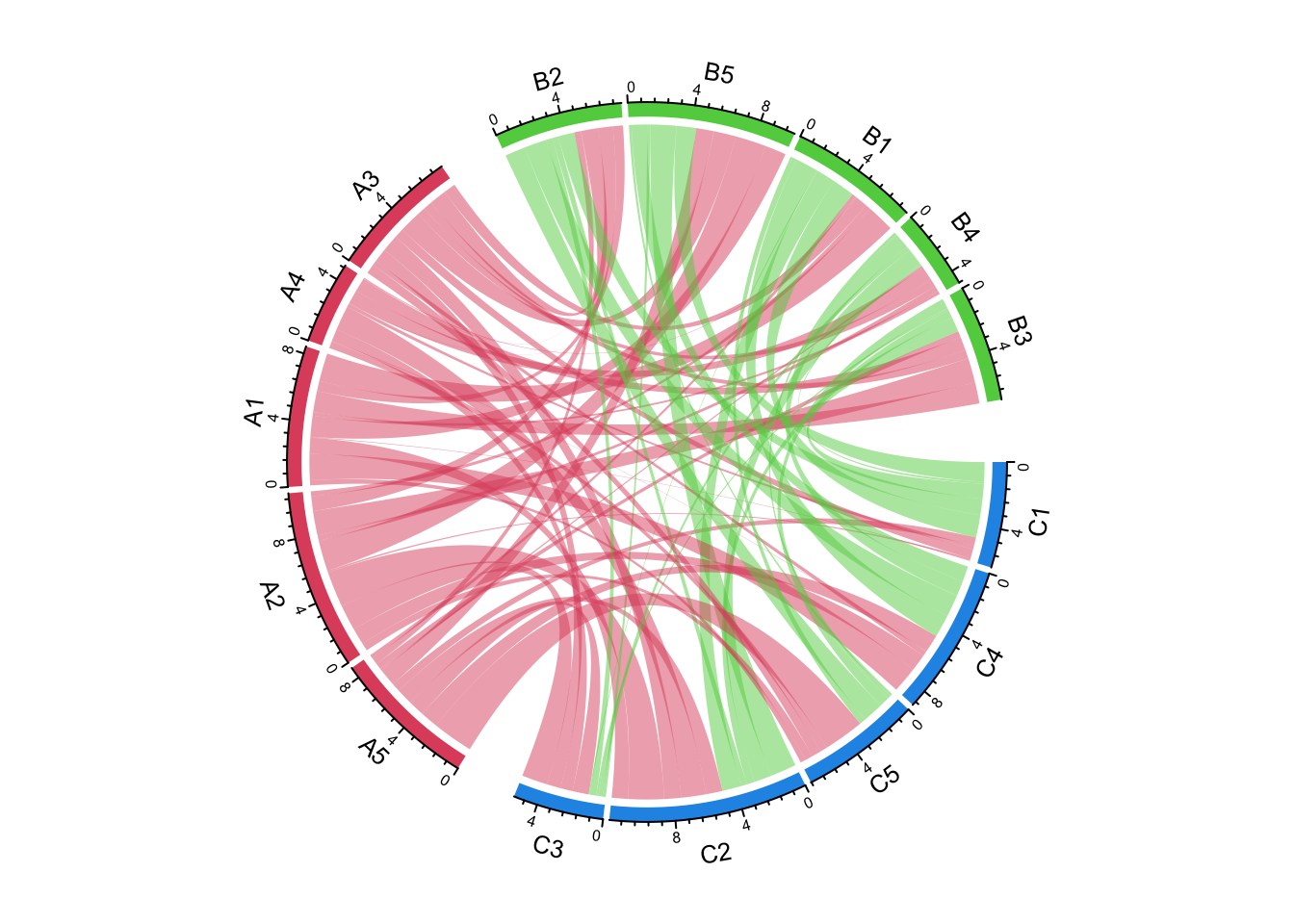circos.clear()

The gap between groups is controlled by big.gap argument and the gap between sectors is controlled by small.gap argument.

group = structure(gsub("\\d", "", nm), names = nm)
chordDiagram(mat, group = group, grid.col = grid.col, big.gap = 20, small.gap = 5)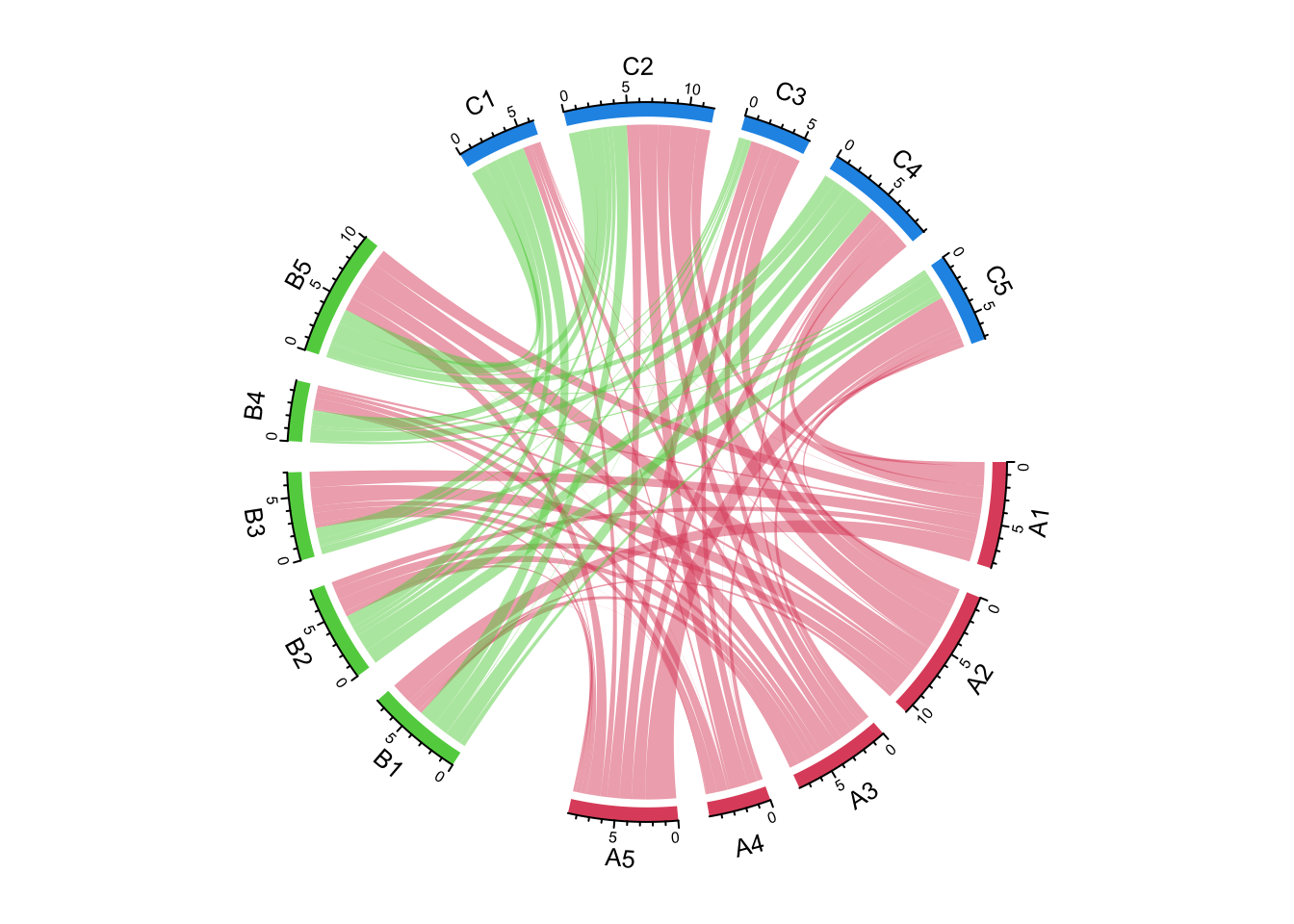circos.clear()

As a normal Chord diagram, the labels and other tracks can be manually adjusted:

group = structure(gsub("\\d", "", nm), names = nm)
chordDiagram(mat, group = group, grid.col = grid.col,
annotationTrack = c("grid", "axis"),
preAllocateTracks = list(
track.height = mm_h(4),
track.margin = c(mm_h(4), 0)
))
circos.track(track.index = 2, panel.fun = function(x, y) {
sector.index = get.cell.meta.data("sector.index")
xlim = get.cell.meta.data("xlim")
ylim = get.cell.meta.data("ylim")
circos.text(mean(xlim), mean(ylim), sector.index, cex = 0.6, niceFacing = TRUE)
}, bg.border = NA)

highlight.sector(rownames(mat1), track.index = 1, col = "red",
text = "A", cex = 0.8, text.col = "white", niceFacing = TRUE)
highlight.sector(colnames(mat1), track.index = 1, col = "green",
text = "B", cex = 0.8, text.col = "white", niceFacing = TRUE)
highlight.sector(colnames(mat2), track.index = 1, col = "blue",
text = "C", cex = 0.8, text.col = "white", niceFacing = TRUE)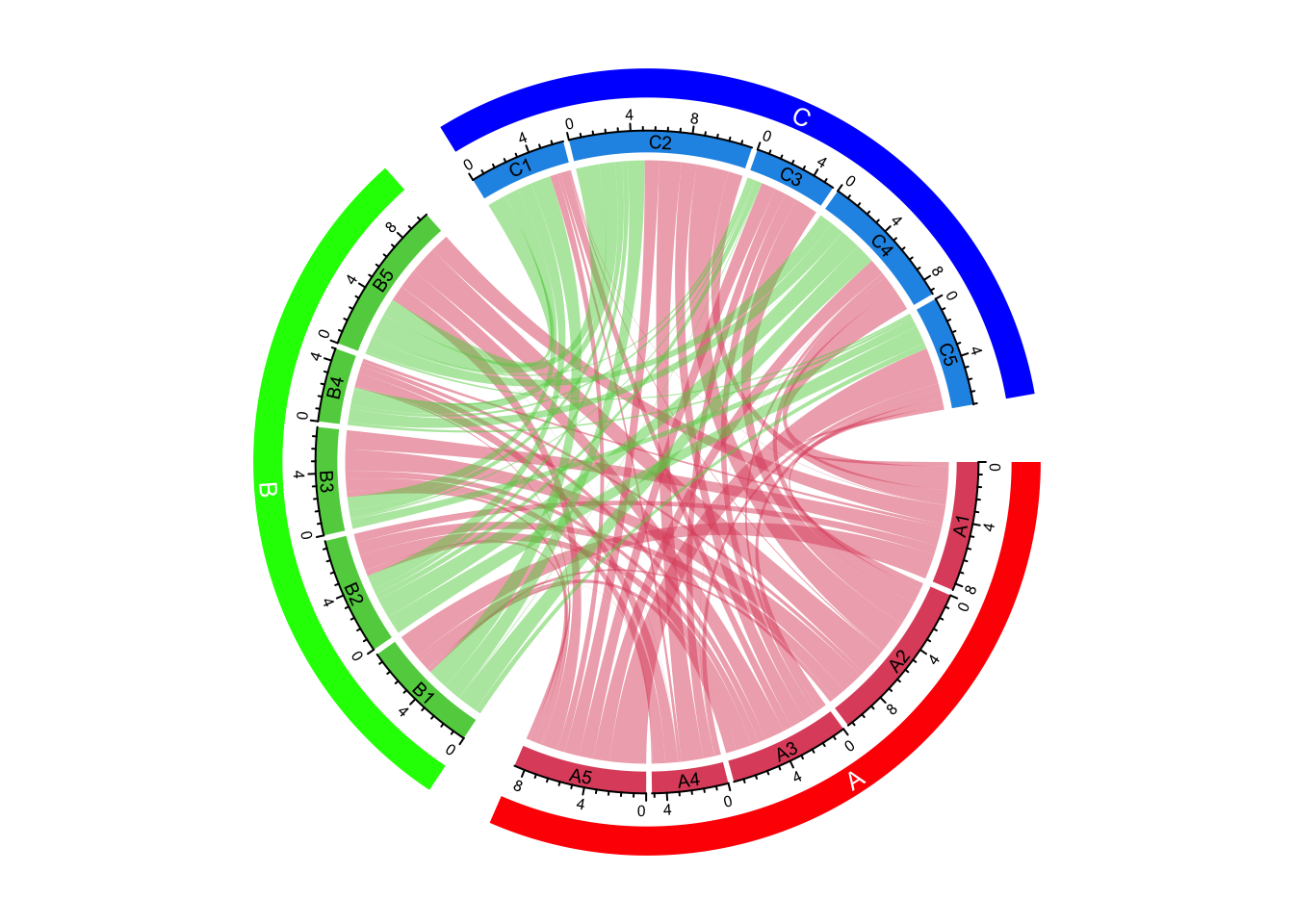circos.clear()